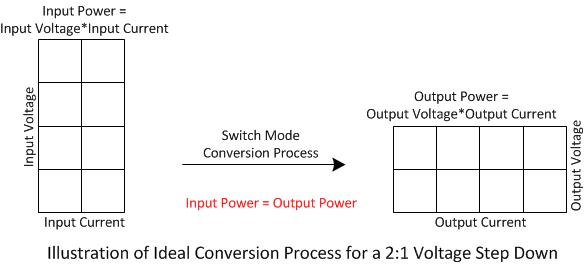# Tutorial: Power Semiconductor Switches | Ideal Switches

This tutorial installment is: Power Semiconductor Switches, Ideal Switches. This topic answers the following questions:

• What is the basic function of a power semiconductor switch in a switching supply?
• What are the most basic properties of a power semiconductor switch?

To view a different topic, go to the Power Supply Tutorial table of contents.
Last topic: Power Supply Capacitors and Inductors
Next topic: Power Semiconductor Switches, Classification

## Power Semiconductor Switch Introduction

All ideal switching supplies continually decompose input power at a given voltage and current into “energy packets”. The small energy packets are then continually reassembled into output power at a new desired voltage and current.

The following image illustrates a 2:1 step down converter where each small block could represent an energy packet:Observe that the output power is equal to that at the input. This property is characteristic of an ideal switching converter. In practical applications there will be some energy loss due to waste heat.

The power semiconductor switches, perform the decomposition and reassembly process, directing the flow of energy from the input, through the inductor and capacitor energy storage components, to the output.

The power semiconductor switches, depending upon the topology and requirements, are either active transistors, thyristors, or passive diode rectifiers. A topology is an arrangement of switches, inductors, and capacitors chosen to achieve a desired input to output conversion ratio.

## Ideal Power Semiconductor Switch Properties

The ideal switch has the following properties:

• infinite breakdown voltage,
• when the switch is off, there is zero current through the switch,
• when switch is on, there is zero voltage across the switch,
• the turn-on and turn-off transition times of ideal switches are zero,
• since the either the voltage or the current is always zero in an ideal switch, the instantaneous dissipation which is the product of instantaneous voltage and instantaneous current is always zero.

## Non-Ideal Power Semiconductor Switch Properties

A well chosen practical switch will approximate an ideal on a first order basis. Even so, the non-ideal properties of practical switches must be evaluated for every design.will have parasitic elements which cause:

• finite breakdown voltage,
• leakage current in the off-state,
• non-zero voltage across the switch in the on-state,
• non-zero turn-on and turn-off transition times,
• since there is some voltage across the switch in the on-state, and the transition times are non-zero, there is non-zero dissipation which must be managed.

## Next Topic

The next tutorial installment is: Power Semiconductor Switches, Classification. This next topic will answer the following questions:

• What are the main power semiconductor switch classifications?
• What are the important properties of the main classifications?

If you need assistance with power electronics design, call or email us today for help with your requirements. You can also go to our power electronics consultant website for more information about our services for business clients. Thank you for reading this tutorial article entitled “Power Semiconductor Switches | Ideal Switches”